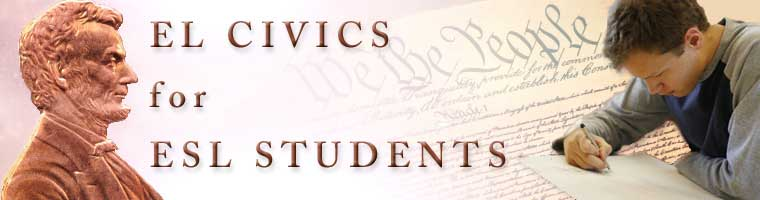# Count by Tens - Page 4

Ninety

ninety = 90

10 + 80 = 90

Ten plus eighty equal ninety.

One-hundred

one-hundred = 100

10 + 90 = 100

Ten plus ninety equal one-hundred.

One-Hundred and Ten

one-hundred and ten = 110

10 + 100 = 110

Ten plus one-hundred equal one-hundred and ten.

110, 120, 130...